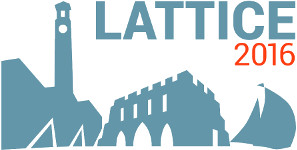#The 34th International Symposium on Lattice Field Theory (Lattice 2016)

Jul 24 – 30, 2016
Highfield Campus, University of Southampton
Europe/London timezone

## Angular and chiral content of the $\rho$ and $\rho^\prime$ mesons

Jul 26, 2016, 3:00 PM
20m
Bulding B2a Room 2065 (Highfield Campus, University of Southampton)

### Speaker

Christian Rohrhofer (University of Graz)

### Description

We identify the chiral and angular momentum content of the leading quark-antiquark Fock component for the $\rho(770)$ and $\rho(1450)$ mesons using a two-flavor lattice simulation with dynamical Overlap Dirac fermions. We extract this information from the overlap factors of two interpolating fields with different chiral structure and from the unitary transformation between chiral and angular momentum basis. For the chiral content of the mesons we find that the $\rho(770)$ slightly favors the $(1,0)\oplus(0,1)$ chiral representation and the $\rho(1450)$ slightly favors the $(1/2,1/2)_b$ chiral representation. In the angular momentum basis the $\rho(770)$ is then a $^3S_1$ state, in accordance with the quark model. The $\rho(1450)$ is a $^3D_1$ state, showing that the quark model wrongly assumes the $\rho(1450)$ to be a radial excitation of the $\rho(770)$.

### Primary author

Christian Rohrhofer (University of Graz)

### Co-authors

Leonid Glozman (University of Graz) Markus Pak (University of Graz)

 Slides